### Consumer’s Equilibrium - Utility & Indifference Curve - Revision Notes

CBSE Class–12 economics

Revision Notes
Micro Economics 02
Consumers Equilibrium & Demand

Consumer : is an economic agent who consumes final goods or services for a consideration.

Utility: is want satisfying power of a commodity.

Total utility: It is the total  satisfaction derived from consumption of given quantity of a commodity at a given time. In other words, It is the sum total of marginal utility.

Marginal Utility: It is the change in total utility resulting from the consumption of an additional unit of the commodity.In other words, It is the utility derived from each additional unit.
Mu= Tun-Tun-1

Relation between total utility and marginal utility

 UNITS Mu Tu 1 10 10 2 8 18 3 6 24 4 4 28 5 2 30 6 0 30 7 -2 28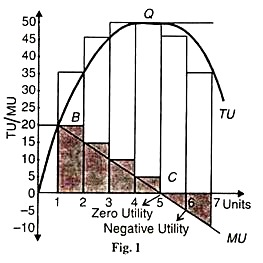1. when Mu diminishes but positive Tu increases at a diminishing rate.
2. when Mu is zero, Tu is maximum.
3. when Mu is negative, Tu diminishes.

Law of Diminishing Marginal Utility : As consumer consumes more and more units of commodity the Marginal utility derived from each successive units go on declining. This is the basis of law of demand.

Consumer’s Bundle :It is a quantitative combination of two goods which can be purchased by a consumer from his given income.

Law of equi-marginal utility- It states that when a consumer spends his income on different commodity he will attain equilibrium or maximize his satisfaction at that point where ratio between marginal utility and price of different commodities are equal and which in turn is equal to marginal utility of money.

Budget set :It is quantitative combination of those bundles which a consumer can purchase from his given income at prevailing market prices.

Consumer Budget :It states the real purchasing power of the consumer from which he can purchase the certain quantitative bundles of two goods at given price.

Budget Line : A graphical representation of all those bundles which cost the amount just equal to the consumers money income gives us the budget line.

Monotonic Preferences :Consumer’s preferences are called monotonic when between any two bundles, one bundle has more of one good and no less of other good as it offers him a higher level of satisfaction.

Change in Budget Line :There can be parallel shift (leftwards or rightwards) due to change in income of the consumer and change in price of goods. A rise in income of the consumer shifts the budget line rightwards and vice-versa.In case of change in price of one good, there will be rotation in the budget line. Fall in price cause outward rotation due to rise in purchasing power and vice-versa.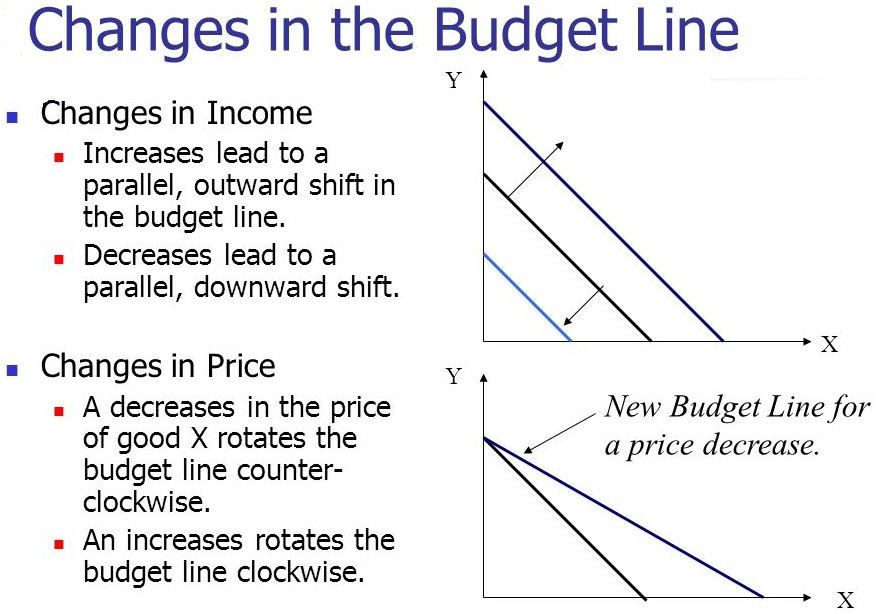Marginal Rate of Substitution (MRS) :It is the rate at which a consumer is willing to substitute (good Y/ good X) one good to obtain one more unit of the other good. Generally, It is the slope of indifference curve.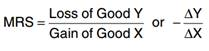Indifference Curve :is a curve showing different combination of two goods, each combinations offering the same level of satisfaction to the consumer.

Characteristics of IC

1. Indifference curves are negatively sloped(i.e. slopes downward from left to right).
2. Indifference curves are convex to the point of origin. It is due to diminishing marginal rate of substitution.
3. Indifference curves never touch or intersect each other. Two points on different IC cannot give equal level of satisfaction.
4. Higher indifference curve represents higher level of satisfaction.

Consumer’s Equilibrium : A consumer is said to be in equilibrium when he maximizes his satisfaction, given his money income and prices of two commodity. He attains equilibrium at that point where the slope of IC is equal to the slope of budget line.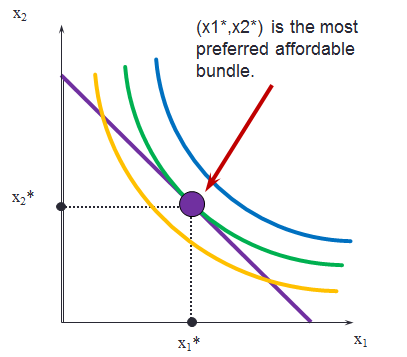Condition of Consumer’s Equilibrium
(a) Cardinal approach (Utility Analysis) :
According to this approach utility can be measured. “Utils” is the unit of utility.

Condition :

1. In case of one community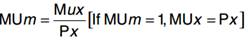Where, MUm = Marginal utility of money
MUx = Marginal utility of ‘x’, Px = Price of ‘x’
2. In case of two commodity.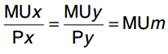xy and MU must be decreasing
 Units MUx MUy MUx/Px MUy/Py 1 36 40 12 10 2 33 36 11 9 3 30 32 10 8 4 27 28 9 7 5 24 24 8 6 6 21 20 7 5

Assumption, Px = Rs.3, Py = Rs.4
Y = Rs.20 Here, MUm = 9

(b) Ordinal approach (Indifference Curve Analysis): According to this approach utility cannot be measured but can be expressed in order or ranking.

Condition of Equilibrium:

1.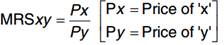or budget line must be tangent to indifference curve.
2. MRS must be diminishing or,
Indifference curve must be convex to the origin.

Quantity Demanded : It is that quantity which a consumer is able and is willing to buy at particular price and in a given period of time.

Determinants of Demand:

1. Price of Good
2. Income of Consumers
3. Taste & Preference of Consumer
4. Change in Price of Related Goods
5. Future Expectation to Change in price

Change of Demand :

1. Change in quantity Demanded or Movement along Demand curve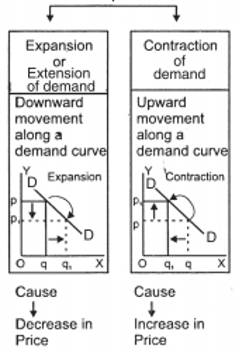2. Change in Demand or Shift in Demand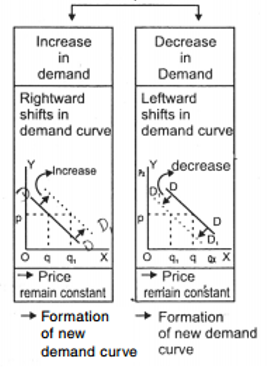Market Demand :It is the total quantity of the commodity demanded in the market by all consumers at different prices at a point of time.

Demand Function :It is the functional relationship between the demand for a commodity and factors affecting demand.

Law of demand :The law states that when all other thing remains constant then there is inverse relationship between price of the commodity and quantity demanded of it. That is, higher the price, lower the demand and lower the price,higher the demand.

Change in Demand : When demand changes due to change in any one of its determinants other than the price.

Change in Quantity Demanded :When demand changes due to change in its own price keeping all other factors constant.

Demand curve and demand schedule : The tabular presentation of price and quantity demanded is called demand schedule and a demand curve is the graphical representation of the demand schedule.

Demand curve and its slope :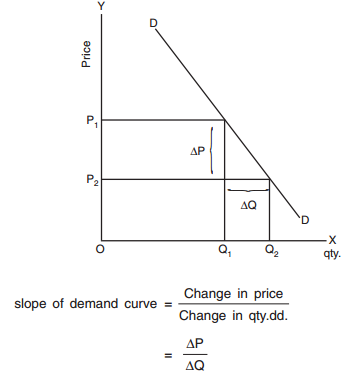Price Elasticity of Demand : Price Elasticity of Demand is a measurement of change in quantity demanded in response to a change in price of the commodity.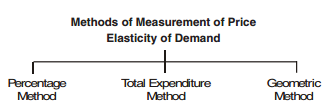Percentage Method :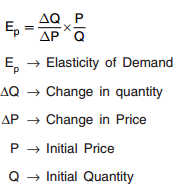Or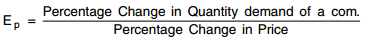Total Expenditure Method : It measures price elasticity of demand on the basis of change in total expenditure incurred on the commodity by a household due to change in its price.
There are three conditions :

1. Ed=1 When due to rise or fall in price of a good, total expenditure remains unchanged.
2. Ed  >1 When due to fall in price, total expenditure goes up and due to rise in price, total expenditure goes down.
3. E<1 when due to fall in price, total expenditure goes down and due to rise in price, total expenditure goes up.

Geometric Method : Elasticity of demand at any point is measured by dividing the length of lower segment of the demand curve with the length of upper segment of demand curve at that point.
The value of ed is unity at mid point of any linear demand curve.

Diagram to show Geometric or point method :
Elasticity of demand at given point.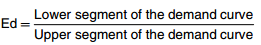D is mid point of the demand curve.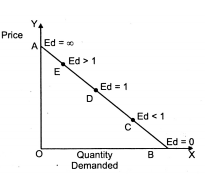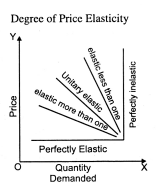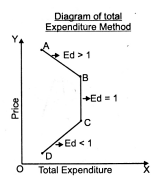Factors influencing Price elasticity of Demand

1. Nature of the Commodity.
2. Availability of Substitute goods.
3. Income level of the consumer.
4. Price level of the commodity.
5. Time Period.
6. Different use of the commodity.
7. Behavior of the consumer.
8. Postponement of consumption.